# Maths formulas for Class 9 CBSE Chapter 3 Coordinate Geometry

Download Maths Formulas For Class 9 CBSE Chapter 3 Coordinate Geometry In Pdf

Chapter 3 Maths formulas for Class 9 CBSE Coordinate Geometry

Constants : A symbol having a fixed numerical value is called a constant.
Example : 7, 3, -2, 3/7, etc. are all constants.

Variables : A symbol which may be assigned different numerical values is known avariable.

Example :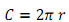cumference of circle
r - radius of circle Where 2 & are constants. while C and r are variable

Algebraic expressions : A combination of constants and variables. Connected by some or all of the operations +, -, X and is known as algebraic expression.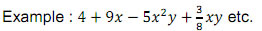Terms : The several parts of an algebraic expression separated by '+' or '-' operations are called the terms of the expression.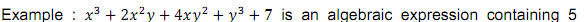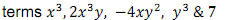Polynomials : An algebraic expression in which the variables involved have only nonnegative integral powers is called a polynomial.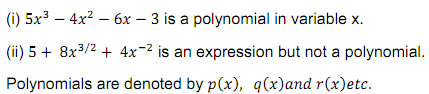Coefficients : In the polynomial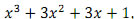coefficient of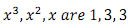respectively and we also say that +1 is the constant term in it. Degree of a polynomial in one variable : In case of a polynomial in one variable the highest power of the variable is called the degree of the polynomial. Classification of polynomials on the basis of degree.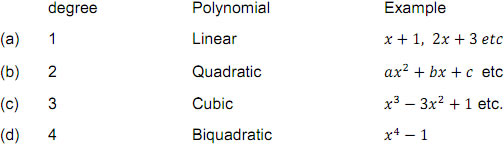Classification of polynomials on the basis of no. of terms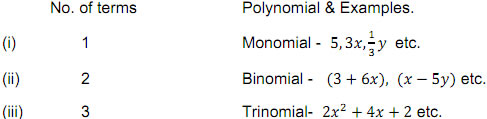Constant polynomial : A polynomial containing one term only, consisting a constanterm is called a constant polynomial the degree of non-zero constant polynomial is zero.

Zero polynomial : A polynomial consisting of one term, namely zero only is called a zero polynomial.The degree of zero polynomial is not defined.
Zeroes of a polynomial : Let p(x).be a polynomial. If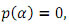then we say that is zero of the polynomial of p(x).

Remark : Finding the zeroes of polynomial p(x) means solving the equation p(x)=0.

Remainder theorem : Let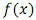be a polynomial of degree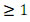and let a be any real number. Whenis divided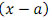by then the remainder is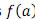Factor theorem : Letbe a polynomial of degree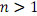and let a be any real number.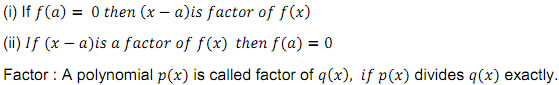Factorization : To express a given polynomial as the product of polynomials each of degree less than that of the given polynomial such that no such a factor has a factor of lower degree, is called factorization.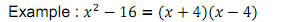Methods of Factorization : Factorization by taking out the common factore.g.Factorization of quadratic trinomials by middle term splitting method.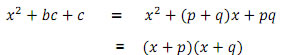Identity : Identity is a equation (trigonometric, algebraic ) which is true for every value of variable.

Some algebraic identities useful in factorization: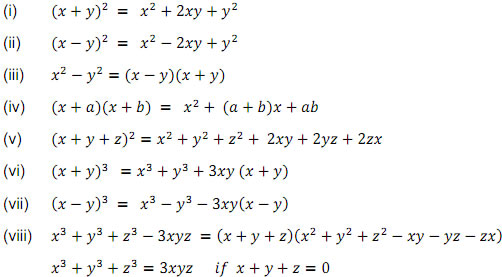### Section - A

Q.1 Which of the following expressions is polynomial?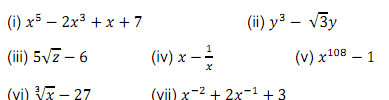Q.2 Write the degree of each of the following polynomial.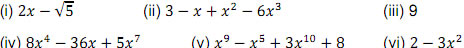Q.3 (i) Give an example of a binomial of degree 27.
(ii) Give an example of a monomial of degree 16.
(iii) Give an example of trinomial of degree 3.

### Section - A

Q.4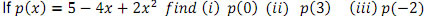Q.5 Find the zeros of the polynomials given below :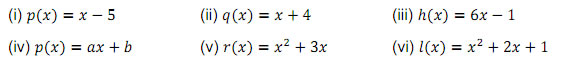Q.6 Find the remainder when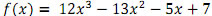is divided by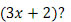Q.8 Find the value of a for which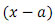is a factor of the polynomial.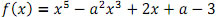### Section - C

Q.9 Factorize the following expressions.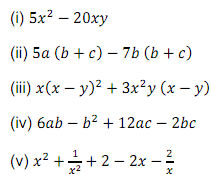Q.10 Factorize :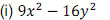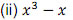Q.11 Factorize: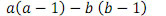Q.12 Factorize following expressions.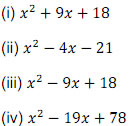Q.13 Calculate

Q.13 Calculate (997)2 using algebraic identities.

Q.14 Calculate 103 X 107 using algebraic identities.

Q.15 Expand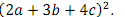Q.16 Factorize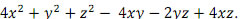Q.17 Expand (i)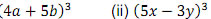Q.18 Evaluate (i)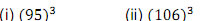Q.19 Factorize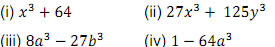### Section - D

Q.20 FactorizeQ.21 Factorize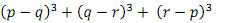Q.22 Find the product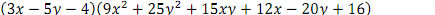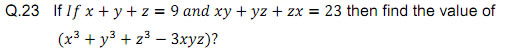### Self Evaluation

Q.24 Which of the following expression is a polynomial?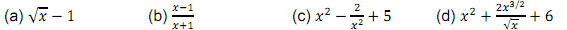Q.25 Degree of zero polynomial is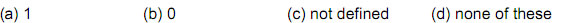Q.26 For what value of k is the polynomial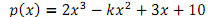exactly divisible by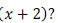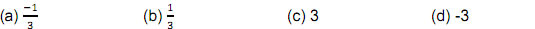Q.27 The zeroes of the polynomial p(x)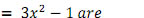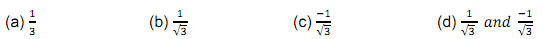Q.28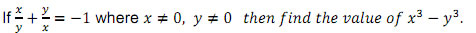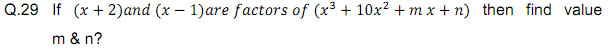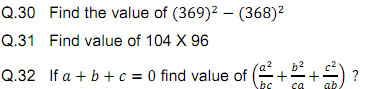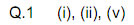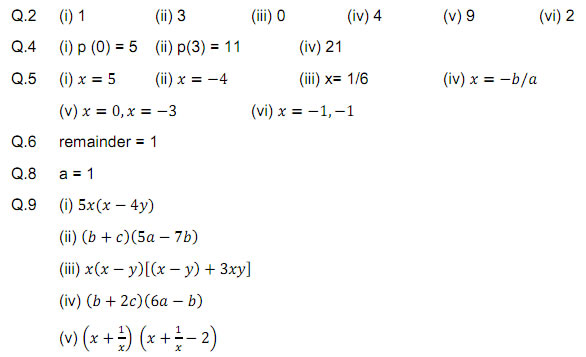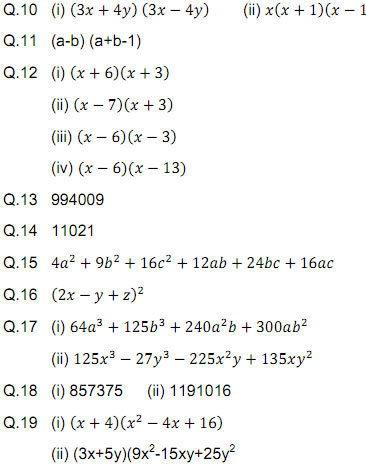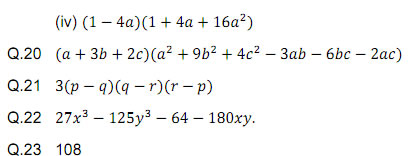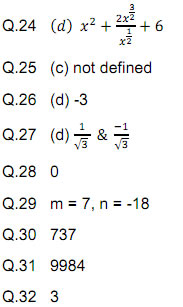### NCERT Books Free Pdf Download for Class 5, 6, 7, 8, 9, 10 , 11, 12 Hindi and English Medium

 Mathematics Biology Psychology Chemistry English Economics Sociology Hindi Business Studies Geography Science Political Science Statistics Physics Accountancy